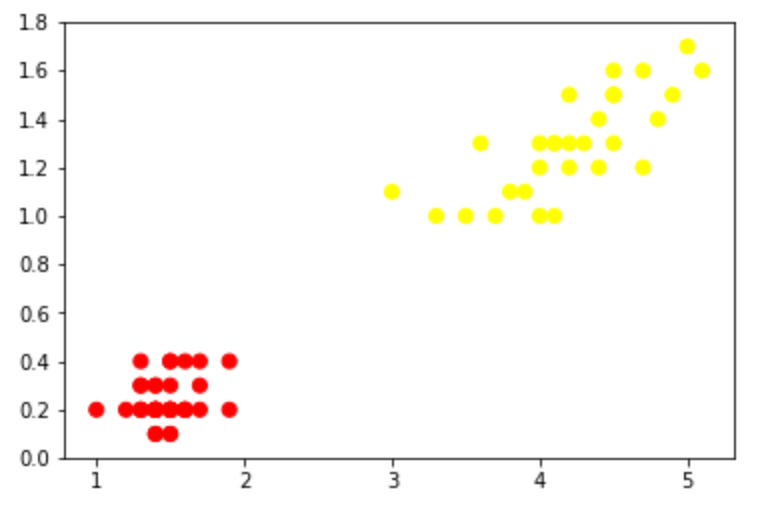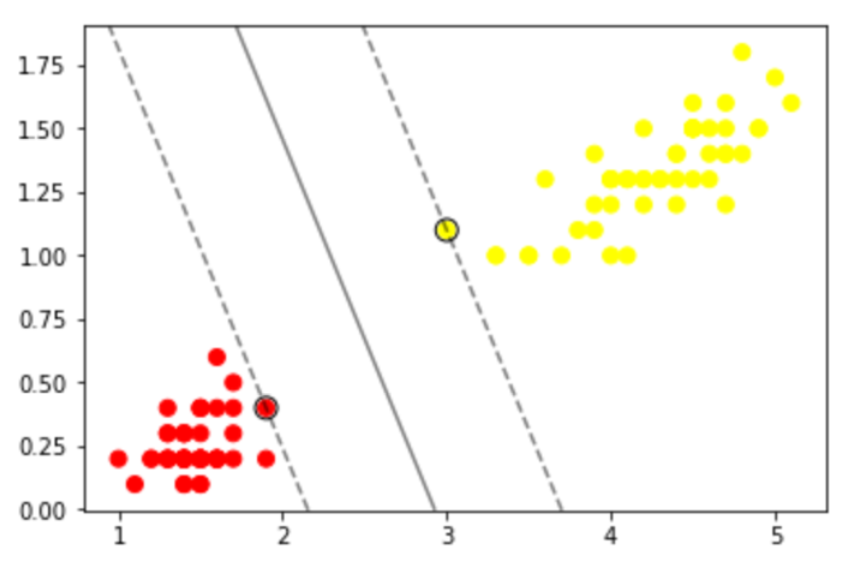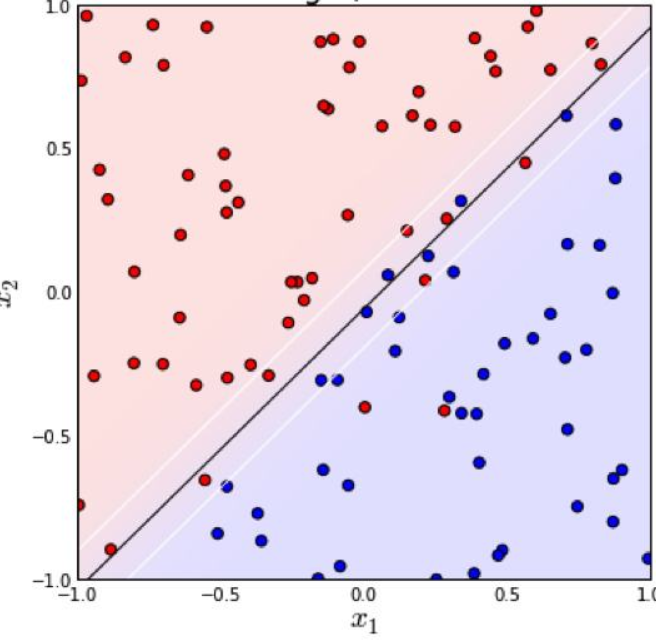# intro

The support-vector mechine is a new learning machine for two-group classification problems. The machine conceptually implements the following idea: input vectors are non-linearly mapped to a very high-dimension feature space. In this feature space a linear decision surface is constructed. Special properties of the decision surface ensures high generalization ability of the learning machine. The idea behind the support-vector network was previously implemented for the restricted case where the training data can be separated without errors. We here extend this result to non-separable training data.

High generalization ability of support-vector networks utilizing polynomial input transformations is demonstrated. We also compare the performance of the support-vector network to various classical learning algorithms that all took part in a benchmark study of Optical Character Recognition.SVM刚诞生时遇到的问题大多都如前者，这样我们直接计算“最大间隔超平面”就ok，这个称为“线性支持向量机”，即最初的SVM；（作者————1963年，万普尼克）

# explanation

1962年提出的线性支持向量机中有两个概念：“硬间隔”，“软间隔”。$\left[{\frac {1}{n}}\sum _{i=1}^{n}\max \left(0,1-y_{i}({\vec {w}}\cdot {\vec {x_{i}}}-b)\right)\right]+\lambda \lVert {\vec {w}}\rVert ^{2},$

# reference

posted @ 2020-11-25 14:33  dynmi  阅读(628)  评论(0编辑  收藏  举报